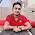# Factorial with C Program

We get factorial of a number by getting the product of the numbers from 1 to that number.  Here is the formula:

factorial(n) or n! = n * (n-1) * (n-2) * …… * 1

So the factorial of 4 will be:
4! = 4 * 3 * 2 * 1 =  24

Here is a C program to get the factorial of a number:

```#include <stdio.h>

int main()
{
int num, i, fact = 1;
printf("Enter a number to know its factorial: ");
scanf("%d",&num);
for(i=num;i>0;i--){
fact = fact * i;
}
printf("Factorial of %d! = %d",num,fact);
return 0;
}
```

Share:

#### 1 comments :

1.Factorial Program in C++

in c++ you can easily write Factorial of any number, it is the product of an integer and all the integers below it for example factorial of 5 is
5! = 5 * 4 * 3 * 2 * 1 = 120. factorial program in c++ is very simple and easy.

Spam comments will be deleted. :)